# KCET 2020 Mathematics Paper with Solutions

KCET 2020 Mathematics question paper and solutions are given here. Students can easily access the solutions from this page. All the solutions are given in step by step method with detailed explanations. These step by step solutions help students to easily understand the problems. The solutions are also available in PDF format which can be downloaded for free. These are prepared by our specialized team of experts and students will find the correct answers to the questions asked in the KCET 2020 Mathematics paper.
With KCET 2020 Mathematics question paper, students can practise expertly and also analyze their performance at the same time. This will also help students to increase their problem-solving speed, which in turn will boost their confidence in the exam hall. The solutions provided here will definitely help students to score higher marks in the upcoming exams.

### KCET 2020 - Maths

1. If

$$y = 2x^{n+1} + \frac{3}{x^n}$$
, then
$$x^2 \frac{d^2y}{dx^2}$$
is

1. a. y
2. b. 6n(n + 1)y
3. c. n(n +1 )y
4. d.
$$x \frac{dy}{dx} + y$$

Solution:

y= (2x)(n+1) +(3x)(-n)

⇒ dy/dx = 2(n+1)xn-(3nx)(-n-1)

⇒ (d2 y)/(dx2) = 2n(n+1)x(n-1)+3n(n+1)x(-n-2)

⇒ x2 (d2 y)/(dx2) = n(n+1) [(2x)(n+1)+3/xn ]

⇒ x2 (d2 y)/(dx2) = n(n+1)y

2. If the curves are 2x = y2 and 2xy = K intersect perpendicularly, then the value of K2 is

1. a. 8
2. b. 4
3. c. 2√2
4. d. 2

Solution:

2x = y2 . . . (1)

2xy = K . . . (2)

Solving (1) and (2), we get

(x,y) = (K(2/3)/2, K(1/3))

Differentiating (1) and (2) w.r.t. x

m1 = dy/dx = 1/y ...(3)

m2 = dy/dx = -y/x ...(4)

∵ Both curves intersect each other perpendicularly

∴m1 m2 = -1

⇒ -1/x = -1

⇒ x = 1

⇒ K(2/3) = 2

⇒ K2 = 8

3. If (xe)y = ex, then dy/dx is

1. a.
$$\frac{e^x}{x(y-1)}$$
2. b.
$$\frac{log \ x}{(1 + log \ x)^2}$$
3. c.
$$\frac{1}{(1 - log \ x)^2}$$
4. d.
$$\frac{log \ x}{1+log \ x}$$

Solution:

(xe)y = ex

⇒y (log x + 1) = x

⇒ y = x/(logx+1)

$$\frac{dy}{dx} = \frac{log \ x}{(log \ x + 1)^2}$$

4. If the side of a cube is increased by 5%, then the surface area of a cube is increased by

1. a. 20%
2. b. 10%
3. c. 60%
4. d. 6%

Solution:

Let one side of the cube be x and surface area be A

So, dx = 5% = 5x/100

Then, A = (6x)2

⇒dA/dx = 12x

⇒dA = (12x)dx

⇒dA = (12x) (5x/100)

⇒dA = 10A/100

⇒dA = 10%

5. The value of

$$\int \frac{1+x^4}{1+x^6}dx$$
is

1. a. tan-1x +(1/3)tan-1x2 + C
2. b. tan-1 x+tan-1x3+C
3. c. tan-1x+(1/3)tan-1 x3+C
4. d. tan-1x-(1/3)tan-1 x3+C

Solution: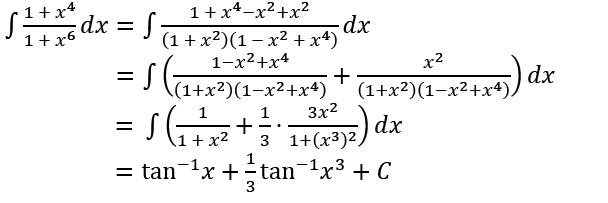6. The maximum value of

$$\frac{log_e \ x}{x}$$
, if x>0 is

1. a. -1/e
2. b. e
3. c. 1
4. d. 1/e

Solution:

$$y = \frac{log_e \ x}{x}$$

$$\frac{dy}{dx}=\frac{1 - log_e x}{x^2}$$

For maxima, dy/dx = 0

⇒ 1 - loge x = 0

⇒ x = e

dy/dx changes sign from positive to negative at x = e

∴ ymax = 1/e

7. The value of

$$\int e^{sin x} sin2x dx$$
is

1. a. 2esin x(cosx-1)+C
2. b. 2esin x(sinx-1)+C
3. c. 2esin x(sinx+1)+C
4. d. 2esin x(cosx+1)+C

Solution:

$$I = \int e^{sinx} \ sin 2x \ dx = 2 \int e^{sinx} \ sin x \ cos x \ dx$$

Let t =sinx

⇒ dt=cosx dx

Therefore, I = 2∫tet dt

= 2(tet - et) + C

= 2(sinx - 1)esin x + C

8. The value of

$$\int^{1/2}_{-1/2} cos^{-1}x \ dx$$
is

1. a. π2/2
2. b. π
3. c. π/2
4. d. 1

Solution: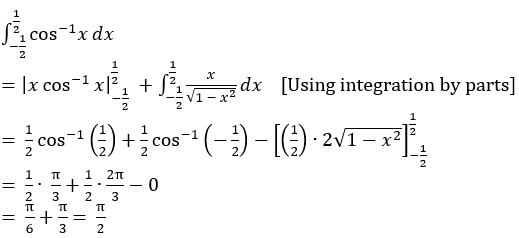9. If

$$\int \frac{3x+1}{(x-1)(x-2)(x-3)}dx = A \ log|x-1|+B \ log|x-2| + C \ log|x-3| + C$$
, then the values of A, B and C are respectively,

1. a. 2, -7, 5
2. b. 5, -7, -5
3. c. 2,-7, -5
4. d. 5, -7, 5

Solution: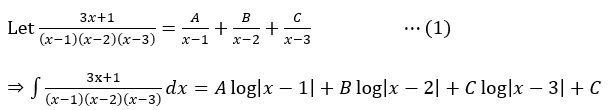Now, 3x + 1 = A(x-2)(x-3) + B(x-1)(x-3) + C(x-1)(x-2) [From eqn. (1)]

Putting x = 1, x = 2, x = 3 in the above equation one at a time, we get

A = 2, B = -7, C = 5.

10. The value of

$$\int_0^1 \frac{log(1+x)}{1+x^2}dx$$
is

1. a. (π/8)log2
2. b. (π/2)log 2
3. c. (π/4)log 2
4. d. 1/2

Solution: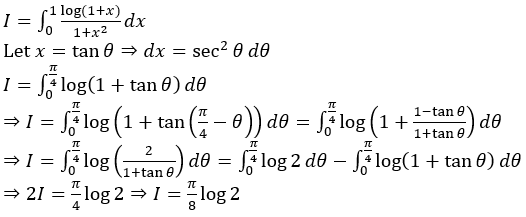11. The area of the region bounded by the curve y2 = 8x and the line y = 2x is

1. a. (8/3) sq. units
2. b. (16/3) sq. units
3. c. (4/3) sq. units
4. d. (3/4) sq. units

Solution:

Solving y2 = 8x and y = 2x, we get

(x,y) = (0,0), (2,4)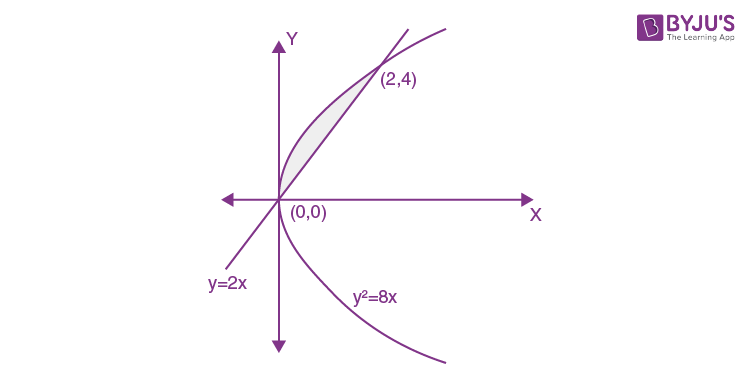So, area bounded by the curve is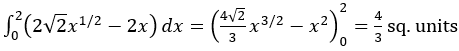12. The value of

$$\int^{\pi/2}_{- \pi/2} \frac{cos \ x}{1 + e^x}dx$$
is

1. a. -2
2. b. 2
3. c. 0
4. d. 1

Solution: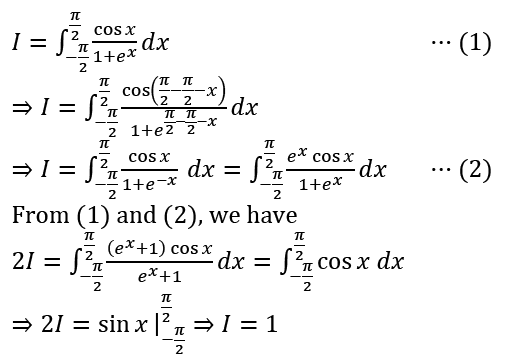13. The order of the differential equation obtained by eliminating arbitrary constants in the family of curves c1y = (c2+c3)e x+c4 is

1. a. 4
2. b. 1
3. c. 2
4. d. 3

Solution:

$$y = [(\frac{C_2 + C_3}{C_1})e^{C_4}]e^x = A e^x,$$

where A =

$$(\frac{C_2 + C_3}{C_1})e^{C_4}$$

Order = Number of independent arbitrary constants = 1

14. The general solution of the differential equation x2dy-2xydx = x4 cos x dx is

1. a. y = cos x+cx2
2. b. y = x2 sin x+cx2
3. c. y = x2sin x+cx
4. d. y = sin x+cx2

Solution:

x2dy - 2xydx = x4 cosx dx

$$\frac{dy}{dx} = \frac{x^4 \ cos x+2xy}{x^2}$$

⇒dy/dx – 2y/x =x2 cos x

I.F. = e∫-2/x dx = e-2 logx = 1/x2

Therefore, the general solution is

$$y(\frac{1}{x^2}) = \int \frac{1}{x^2}(x^2 \ cos x)dx = sinx + c$$

∴y = x2 (sinx+c)

= x2 sinx+cx2

15. The area of the region bounded by the line y = 2x+1, x- axis and the ordinates x = -1 and x = 1 is

1. a. 5
2. b. 9/4
3. c. 2
4. d. 5/2

Solution: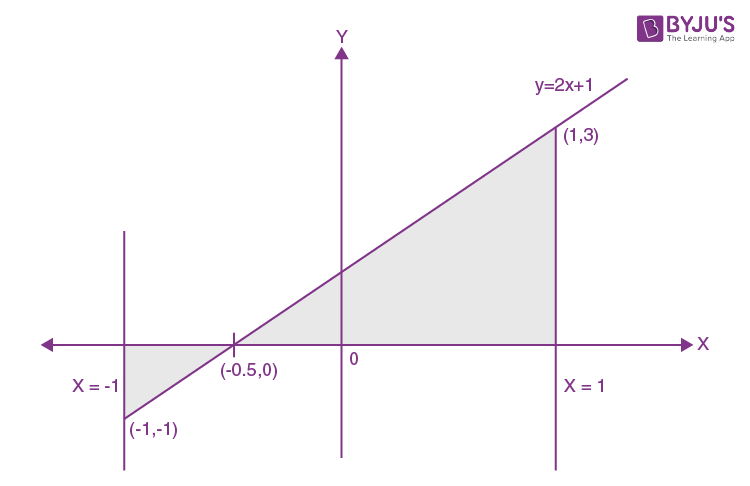Area bounded by y = 2x+1 with x- axis

= (1/2) (1/2)(1) +(1/2) (3/2)(3) = 5/2 sq. units.

16. The two vectors

$$\hat i + \hat j + \hat k$$
and
$$\hat i + 3 \hat j + 5\hat k$$
represent the two sides
$$\vec {AB}$$
and
$$\vec {AC}$$
respectively of a ∆ABC. The length of the median through A is

1. a. 14
2. b. 14/2
3. c. 14
4. d. 7

Solution: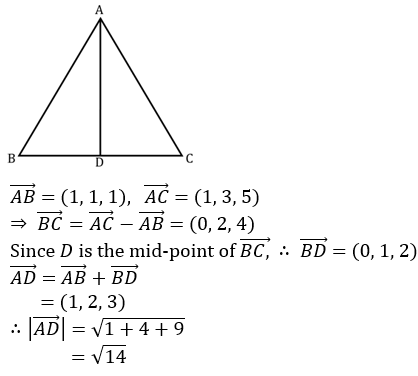17. If

$$\vec {a}$$
and
$$\vec {b}$$
are unit vectors and θ is the angle between
$$\vec {a}$$
and
$$\vec {b}$$
, then sin(θ/2)is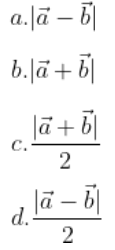Solution: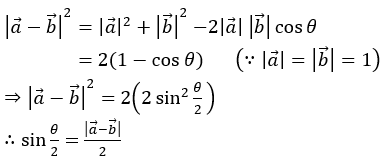18. The curve passing through the point (1, 2) given that the slope of the tangent at any point (x,y) is 2x/y represents

1. a. Hyperbola
2. b. Circle
3. c. Parabola
4. d. Ellipse

Solution:

Given,

$$\frac{dy}{dx}= \frac{2x}{y}$$

⇒ ydy = 2xdx

⇒ ∫ydy = ∫2x dx

⇒ y2/2 = x2+A, where A is a constant.

The above equation represents a hyperbola.

19. If

$$\left | \vec{a} \times \vec{b} \right |^{2} + \left | \vec{a} \cdot \vec{b} \right |^{2}$$
and
$$|\vec a|$$
= 6, then
$$\left | \vec{b} \right |$$
is equal to

1. a. 4
2. b. 6
3. c. 3
4. d. 2

Solution: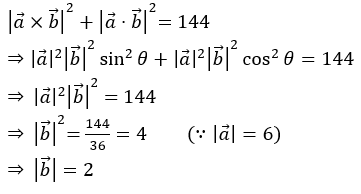20. The point(1,-3,4) lies in the octant

1. a. Eighth
2. b. Second
3. c. Third
4. d. Fourth

Solution:

Signs of x-coordinate, y-coordinate and z-coordinate are +,-,+ respectively.

∴(1,-3,4) lies in the fourth octant.

21. If the vector

$$2 \hat i-3 \hat j + 4 \hat k$$
,
$$2 \hat i+ \hat j - \hat k$$
and
$$\lambda \hat i- \hat j + 2 \hat k$$
are coplanar, then the value of λ is

1. a. 5
2. b. 6
3. c. -5
4. d. -6

Solution:

Given vectors are coplanar.

$$\begin{vmatrix} 2 &-3 &4 \\ 2& 1 &-1 \\ \lambda & -1 &2 \end{vmatrix} = 0$$

⇒ 2(1) + 3(4 + λ) + 4(-2 - λ) = 0

⇒ 2 + 12 + 3λ - 8 - 4λ = 0

⇒ λ = 6

22. The distance of the point (1,2,-4) from the line (x-3)/2 = (y-3)/3 = (z+5)/6 is

1. a. √293/49
2. b. 293/7
3. c. √293/7
4. d. 293/49

Solution:

Let

$$\frac{x-3}{2}=\frac{y-3}{3}=\frac{z+5}{6}=t$$

⇒ (x, y, z) = (2t + 3, 3t + 3, 6t - 5)

∴ d.r.’s of the line perpendicular to

$$\frac{x-3}{2}=\frac{y-3}{3}=\frac{z+5}{6}$$
and joining (2t+3,3t+3,6t-5)

and (1,2,-4) is (2t+2,3t+1,6t-1)

∴2(2t+2)+3(3t+1)+6(6t-1)=0

⇒t = -1/49

∴ Distance =

$$\sqrt{(2t+2)^2+(3t+1)^2+(6t-1)^2} = \sqrt{49t^2+2t+6}$$

=

$$\sqrt{\frac{1}{49}-\frac{2}{49}+6}=\frac{\sqrt{293}}{7}$$

23. The sine of the angle between the straight line (x-2)/3 = (3-y)/(-4) = (z-4)/5 and the plane

1. a. √2/10
2. b. 3/√50
3. c. 3/50
4. d. 4/5√2

Solution:

Given line is

$$\frac{x-2}{3}=\frac{y-3}{4}=\frac{z-4}{5}$$

and plane is 2x-2y+z = 5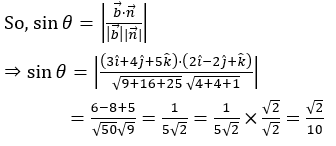24. If a line makes an angle of π/3 with each of x and y-axis, then the acute angle made by z-axis is

1. a. π/2
2. b. π/4
3. c. π/6
4. d. π/3

Solution:

Given, α = β = π/3

Let acute angle made by z- axis be γ.

Then,

$$cos^2 \alpha + cos^2 \beta + cos^2 \gamma$$

$$(\frac{1}{2})^2+(\frac{1}{2})^2 + cos^2 \gamma = 1$$

$$\frac{1}{4}+\frac{1}{4} + cos^2 \gamma = 1 ⇒ cos^2 \gamma = \frac{1}{2}$$

$$cos \gamma = \pm \frac{1}{\sqrt 2}⇒ \gamma = \frac{\pi}{4}$$

[∵γ is acute]

25. Corner points of the feasible region determined by the system of linear constraints are (0,3),(1,1) and (3,0). Let z = px+qy, where p,q>0. Condition on p and q so that the minimum of z occurs at (3,0) and (1,1) is

1. a. p = q
2. b. p = 2q
3. c. p = q/2
4. d. p = 3q

Solution:

Given corner points are (0,3),(1,1),(3,0)

z = px + qy

At (3,0), z = 3p

At (1,1), z = p + q

It is given that the minimum of z occurs at (3, 0) and (1, 1)

⇒ 3p = p + q

⇒ 2p = q

⇒ p = q/2

26. The feasible region of an LPP is shown in the figure. If Z = 11x+7y, then the maximum value of Z occurs at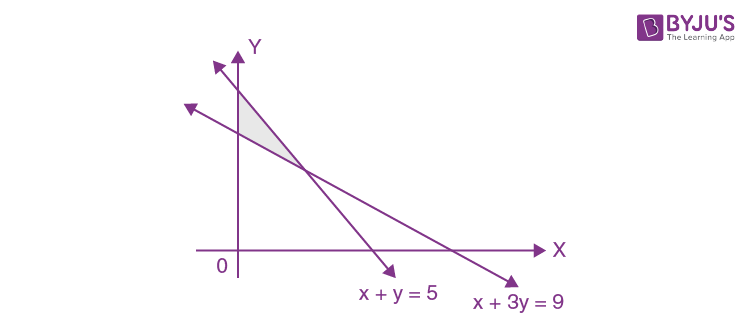1. a. (3,2)
2. b. (0,5)
3. c. (3,3)
4. d. (5,0)

Solution:

y-intercept of x+y = 5 is (0,5)

y-intercept of x+3y = 9 is (0,3)

The intersection point of x+y = 5 and x+3y = 9 is (3,2)

Therefore, the corner points are (0,5),(0,3),(3,2)

At (0,5), Z = 35

At (0,3), Z = 21

At (3,2), Z = 47

So, Zmax= 47 at (3,2).

27. A die is thrown 10 times, the probability that an odd number will come up atleast one time is

1. a. 1013/1024
2. b. 1/1024
3. c. 1023/1024
4. d. 11/1024

Solution:

Given n = 10

Probability of odd number, p = ½

∴q=1/2

Required probability = P(X≥1)

=1-P(X=0)

=

$$1-^{10}C_0 (1/2)^{10-0}(1/2)^0$$

= 1 – 1/210

= 1 - 1/1024

= 1023/1024

28. If A and B are two events such that P(A) = 1/3, P(B) = 1/2 and P(A∩B) = 1/6, then P(A'|B) is

1. a. 1/12
2. b. 2/3
3. c. 1/3
4. d. 1/2

Solution:

Given P(A) = 1/3, P(B) = 1/2, P(A ∩ B) = 1/6

So, P(A’|B) = 1 – P(A|B)

=

$$1 - \frac{P(A \cap B)}{P(B)}$$

= 1 - 1/3

= 2/3

29. Events E1 and E2 form a partition of the sample space S.A is any event such that P(E1) = P(E2) = 1/2, P(E2│A) = 1/2 and (A│E2) = 2/3 . Then P(E1│A) is

1. a. 1/4
2. b. 1/2
3. c. 2/3
4. d. 1

Solution:

Let P(A|E1) = x

By Bayes’ theorem,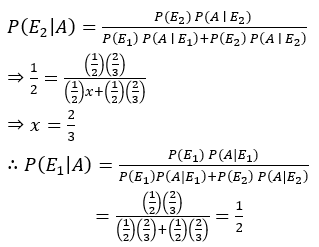30. The probability of solving a problem by three persons A,B and C independently is 1/2,1/4 and 1/3 respectively. Then the probability that the problem is solved by any two of them is

1. a. 1/8
2. b. 1/12
3. c. 1/4
4. d. 1/24

Solution:

Required probability = P(A'BC) + P(AB'C) + P(ABC')

= 1/2 × 1/4 × 1/3 + 1/2 × 3/4 × 1/3 + 1/2 × 1/4 × 2/3

= 1/24 + 1/8 + 1/12

=(1+3+2)/24

= 1/4

31. If n(A) = 2 and total number of possible relations from set A to set B is 1024, then n(B) is.

1. a. 5
2. b. 512
3. c. 20
4. d. 10

Solution:

n(A) = 2

Given, 2(n(A)⋅n(B)) = 1024

⇒(2)(2⋅n(B)) = (2)10

⇒2⋅n(B) = 10

⇒n(B) = 5

32. The value of sin2 510 + sin2 390 is

1. a. cos 120
2. b. 1
3. c. 0
4. d. sin 120

Solution:

sin2 51°+sin2 39°

= cos2 39° + sin2 39°

= 1

33. If tan A+cot A=2, then the value of tan4 A+cot4 A=

1. a. 5
2. b. 2
3. c. 1
4. d. 4

Solution:

tan A + cot A = 2

⇒ (tan A + cotA )2 = 4

⇒tan2 A + cot2 A + 2tan A cotA = 4

⇒tan2 A+cot2 A=2

⇒(tan2 A+cot2 A) 2=4

⇒tan4 A+cot4 A+2 tan2 A cot2 A=4

⇒tan4 A+cot4 A=2

34. If A={1,2,3,4,5,6}, then the number of subsets of A which contain atleast two elements is

1. a. 58
2. b. 64
3. c. 63
4. d. 57

Solution:

Total number of subsets of A is 2n(A) = 26 = 64

Number of subsets of A which contain at least two elements is

64-( 6C0 + 6C1 )

= 64-(1+6)

= 57

35. If z = x + iy, then the equation |z+1| = |z-1| represents

1. a. y-axis
2. b. a circle
3. c. a parabola
4. d. x-axis

Solution: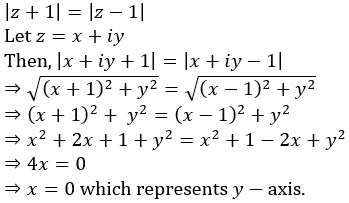36. The value of 16 C9+16 C10- 16C6- 16C7 is

1. a. 17C2
2. b. 0
3. c. 1
4. d. 17C10

Solution:

16C9+ 16C10- 16C6- 16C7

= 16C9+ 16C10- 16C10- 16C9=0 (∵ nCr = nC(n-r))

37. The number of terms in the expansion of (x+y+z)10 is

1. a. 110
2. b. 66
3. c. 142
4. d. 11

Solution:

Number of terms in the expansion of (x+y+z)10 is (10+3-1)C10

= 12C10=12!/(2! 10!)=66

38. If P(n) ∶2n< n!,then the smallest positive integer for which P(n)is true if

1. a. 5
2. b. 2
3. c. 3
4. d. 4

Solution:

For n = 1, 2, 3, 2n> n!

P(4) ∶ 24< 4!

So, smallest positive integer, n = 4.

39. The two lines lx+my = n and l'x+m'y= n’ are perpendicular if

1. a. lm' + ml'= 0
2. b. ll'+mm'=0
3. c. lm'=ml'
4. d. lm+l'm'=0

Solution:

Product of slopes = -1

⇒ll’ + mm’ = 0

40. If the parabola x2 = 4ay passes through the point (2, 1), then the length of the latus rectum is

1. a. 8
2. b. 1
3. c. 4
4. d. 2

Solution:

x2=4ay

Given parabola passes through (2,1).

⇒ 22= 4a

⇒ a = 1

Length of latus rectum= 4a = 4.

41. If the sum of n terms of an A.P. is given by Sn = n2 + n, then the common difference of the A.P. is

1. a. 6
2. b. 4
3. c. 1
4. d. 2

Solution:

Sn= n2+n

S1 = 1+1 = 2 = T1

S2 = 22+2 = 6 = T1+T2

∴T2 = S2-S1= 4

Common difference, d= T2-T1

= 4-2

= 2

42. The negation of the statement “For all real numbers x and y, x + y = y + x” is

1. a. for some real numbers x and y, x - y = y - x
2. b. for all real numbers x and y, x+y ≠ y+x
3. c. for some real numbers x and y, x+y = y+x
4. d. for some real numbers x and y,x+y ≠ y+x

Solution:

Negation: For some real numbers x and y, x + y ≠ y + x.

43. The standard deviation of the data 6,7,8,9,10 is

1. a. 10
2. b. 2
3. c. 10
4. d. 2

Solution:

Mean,

$$\bar{x} = \frac{6+7+8+9+10}{5} = \frac{40}{5} = 8$$

Standard deviation,

$$\sigma = \sqrt{\frac{1}{5}(4+1+0+1+4)}$$

Because,

$$\sigma = \sqrt{\frac{1}{n} \sum(x_i - \bar{x})^2}$$

$$\sigma = \sqrt{\frac{10}{5}} = \sqrt{2}$$

44.

$$lim_{x \rightarrow 0}(\frac{tan \ x}{\sqrt{2x+4}-2})$$
is equal to

1. a. 6
2. b. 2
3. c. 3
4. d. 4

Solution: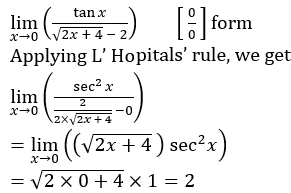45. If a relation R on the set {1,2,3} be defined by R = {(1,1)}, then R is

1. a. Only symmetric
2. b. Reflexive and symmetric
3. c. Reflexive and transitive
4. d. Symmetric and transitive

Solution:

R={(1,1)} on set {1,2,3}

Clearly, R is symmetric and transitive.

46. Let f ∶ [2, ∞] → R be the function defined by f(x) = x2-4x+5, then the range of f is

1. a. [5,∞)
2. b. (-∞,∞)
3. c. [1, ∞)
4. d. (1,∞)

Solution:

f(x) = (x-2)2 + 1 ≥ 1, ∀ x ∈ [2,∞)

fmin = 1 at x = 2

∴ Range of f is [1, ∞)

47. If A,B,C are three mutually exclusive and exhaustive events of an experiment such that P(A) = 2P(B) = 3P(C), then P(B) is equal to

1. a. 4/11
2. b. 1/11
3. c. 2/11
4. d. 3/11

Solution:

Given, P(A) = 2P(B) = 3P(C)

⇒ P(C) = 2/3 P(B)

Since A,B,C are three mutually exclusive and exhaustive events

∴P(A)+P(B)+P(C) = 1

⇒ P(B) = 3/11

48. The domain of the function defined by f(x) = cos-1 √(x-1)is

1. a. [0,1]
2. b. [1,2]
3. c. [0,2]
4. d. [-1,1]

Solution:

For f to be defined,

x -1 ≥ 0 and -1 ≤ √(x-1) ≤ 1

⇒ x ≥ 1 and 0 ≤ x-1 ≤ 1

⇒ x ≥ 1 and 1 ≤ x ≤ 2

⇒ x ∈ [1,2]

Hence, domain of f is [1, 2].

49. The value of

$$cos(sin^{-1} \frac{\pi}{3} + cos^{-1} \frac{\pi}{3})$$
is

1. a. Does not exist
2. b. 0
3. c. 1
4. d. -1

Solution:

π/3 ∉ [-1,1] which is the domain of sin-1 x, cos-1 x

So, cos(sin-1 π/3 + cos-1 π/3) does not exist.

50. If

$$\begin{pmatrix} 0 & 0 & 1 \\ 0 & 1 &0 \\ 1&0 &0 \end{pmatrix}$$
, then A4 is equal to

1. a. 4A
2. b. A
3. c. 2A
4. d. I

Solution: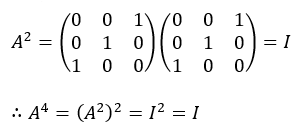51. If A = {a,b,c}, then the number of binary operations on A is

1. a. 39
2. b. 3
3. c. 36
4. d. 33

Solution:

A = {a,b,c}

n(A) = 3

Number of binary operations is n(A)(n(A))^2 = 3(3^2) = 39

52. If

$$\begin{pmatrix} 2 &1 \\ 3& 2 \end{pmatrix}A = \begin{pmatrix} 1 &0 \\ 0 &1 \end{pmatrix}$$
, then the matrix A is

1. a.
$$\begin{pmatrix} 2 &-1 \\ 3& 2 \end{pmatrix}$$
2. b.
$$\begin{pmatrix} 2 &1 \\ 3& 2 \end{pmatrix}$$
3. c.
$$\begin{pmatrix} 2 &-1 \\ -3& 2 \end{pmatrix}$$
4. d.
$$\begin{pmatrix} -2 &1 \\ 3&- 2 \end{pmatrix}$$

Solution: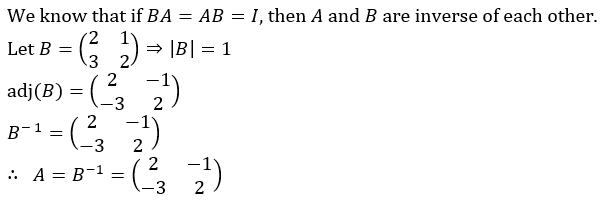53. If f(x) =

$$\begin{vmatrix} x^3-x &a+x&b+x\\ x-a & x^2-x &c+x \\ x-b &x-c& 0 \end{vmatrix}$$
, then

1. a. f(-1) = 0
2. b. f(1) = 0
3. c. f(2) = 0
4. d. f(0) = 0

Solution:

$$f(0) = \begin{vmatrix} 0& a& b\\ -a& 0&c \\-b & -c& 0\end{vmatrix}$$

f(0) is the determinant of skew-symmetric matrix of order 3 (odd).

∴f(0) = 0.

54. If A and B are square matrices of same order and B is a skew symmetric matrix, then A’BA is

1. a. Skew symmetric matrix
2. b. Symmetric matrix
3. c. Null matrix
4. d. Diagonal matrix

Solution:

B is a skew symmetric matrix.

⇒ B' = -B

Now, (A'BA)' = A'B'(A')'

= A'(-B)A

= -(A' BA)

Hence, A'BA is a skew symmetric matrix.

55. If A is a square matrix of order 3 and |A| = 5, then |A adj A| is

1. a. 625
2. b. 5
3. c. 125
4. d. 25

Solution:

= |A||A|3-1

= 5 52

= 125

56. If

$$f(x) = \left\{\begin{matrix} \frac{1-cos \ kx}{x \ sin x} & if x \neq 0 \\ \frac{1}{2} & if x = 0 \end{matrix}\right.$$
is continuous at x = 0, then the value of K is

1. a. ±1
2. b. ±1/2
3. c. 0
4. d. ±2

Solution:

Given, f is continuous at x = 0.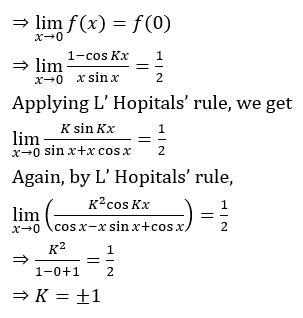57. If a1,a2,a3,……,a9are in A.P. then the value of

$$\begin{vmatrix} a_1 &a_2 &a_3 \\ a_4&a_5 &a_6 \\ a_7& a_8 &a_9 \end{vmatrix}$$
is

1. a. 1
2. b. (9/2)(a1+a9)
3. c. a1+a9
4. d. loge(logee)

Solution: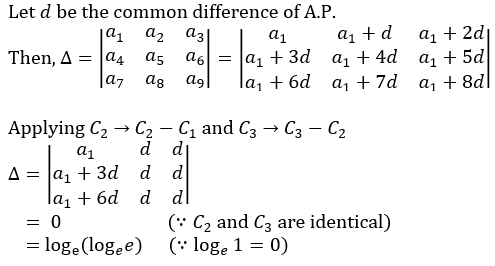58. If 2x + 2y = 2(x+y), then dy/dx is

1. a.
$$\frac{2^y-1}{2^x-1}$$
2. b. 2y-x
3. c. -2y-x
4. d. 2x-y

Solution:

2x + 2y = 2(x+y).....(1)

Differentiating both sides w.r.t. x, we get

2x ln 2 + 2y y' ln2 = 2(x+y) ln2 (1+y')

⇒2x+2y y'=2(x+y)(1+y')

⇒2x+2y⋅y'= 2(x+y)+2(x+y)⋅y'

⇒2x-2(x+y)=y'(2(x+y)-2y)

⇒2x-2x-2y=y'(2x+2y-2y) [From eqn.(1)]

⇒-2y=y'2x

⇒y'= dy/dx=-2y-x

59. If

$$f(x) = sin^{-1}(\frac{2x}{1+x^2})$$
, then f’(√3)is

1. a. -1/√3
2. b. -1/2
3. c. 1/2
4. d. 1/√3

Solution: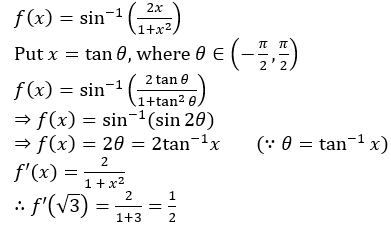60. The right hand and left limit of the function

$$f(x) = \left\{\begin{matrix} \frac{e^{1/x}-1}{e^{1/x}+1} & if \ x \neq 0\\ 0 & if \ x = 0 \end{matrix}\right.$$
are respectively

1. a. -1 and 1
2. b. 1 and 1
3. c. 1 and -1
4. d. -1 and -1

Solution: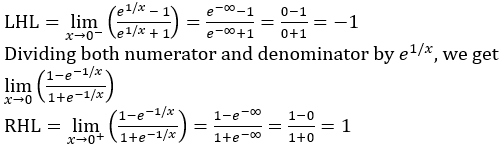### KCET 2020 Maths Question Paper with Solutions